# The Variety of Demand Curves

## Introduction

• The price elasticity of demand is related to the slope of the demand curve.
• Rule of thumb:
The flatter the curve, the bigger the elasticity.
The steeper the curve, the smaller the elasticity.
• Five different classifications of D curves.

### Perfectly Inelastic Demand

Price of elasticity of demand = (Percentage change in Q) / (Percentage change in P) =
0% / 15% = 0

 D curve: Vertical Consumers' price sensitivity: None Elasticity: 0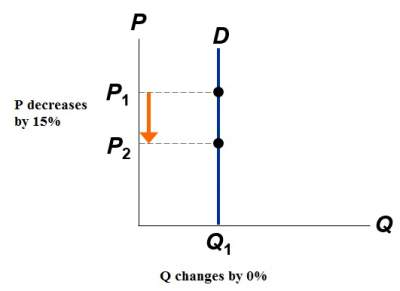### Inelastic Demand

Price of elasticity of demand = (Percentage change in Q) / (Percentage change in P) = (< 15%) / (15%) < 1
 D curve: relatively steep Consumers' price sensitivity: relatively low Elasticity: < 1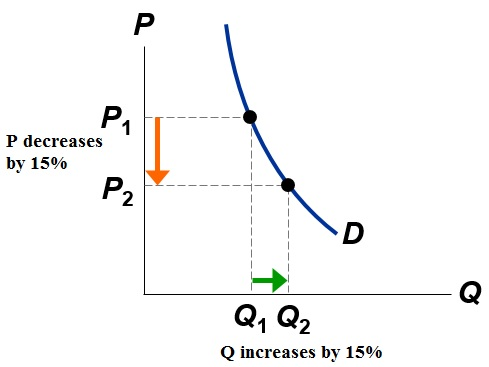### Unit Elastic Demand

Price of elasticity of demand = (Percentage change in Q) / (Percentage change in P) = (15%) / (15%) = 1
 D curve: intermediate slope Consumers' price sensitivity: intermediate Elasticity: = 1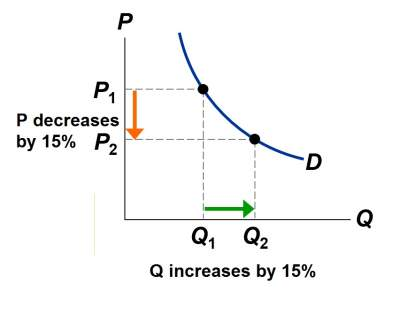### Elastic Demand

Price of elasticity of demand = (Percentage change in Q) / (Percentage change in P) = (> 15%) / (15%) > 1
 D curve: relatively flat Consumers' price sensitivity: relatively high Elasticity: > 1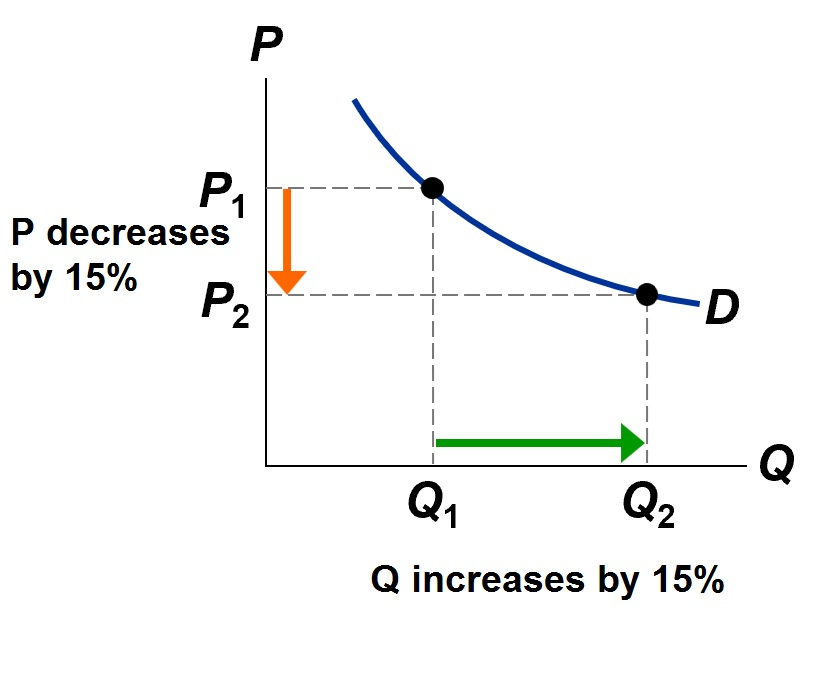### Perfectly Elastic Demand

Price of elasticity of demand = (Percentage change in Q) / (Percentage change in P) = (any value) / (0%) = ∞
 D curve: horizontal Consumers' price sensitivity: extreme Elasticity: ∞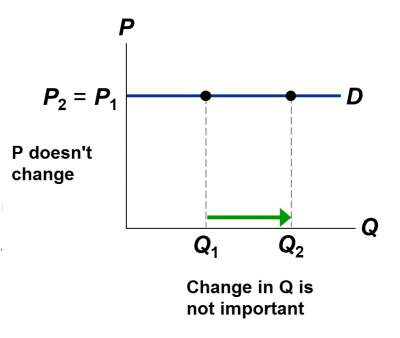### Elasticity of a Linear Demand Curve

The slope of a linear demand curve is constant, however its elasticity is not as illustrated below.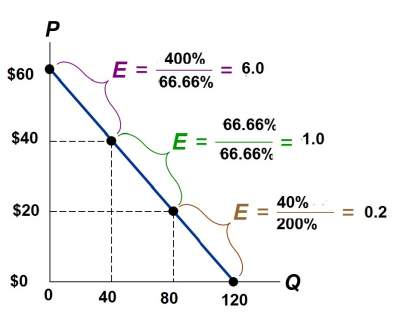### Price Elasticity and Total Revenue

• As illustrated in the previous example, where if price is increased from \$250 to \$350, and the question is: would your revenue rise or fall?
The formula is: Revenue = P × Q
• A price increase has two effects on revenue:
Higher price (P) means that we should expect increase in the revenue on each unit sold.
However, whenever fewer units are sold leading to a decrease in quantity (Q), due to Law of Demand.
• Which of these two effects is more important?
The answer is that it all depends on the price elasticity of demand.
Price of elasticity of demand = (Percentage change in Q) / (Percentage change in P)
Revenue = P × Q
• If demand is elastic, then price elasticity of demand > 1
• % change in Q > % change in P
• The fall in revenue from lower Q is greater than the increase in revenue from higher P, hence revenue falls.
 Elastic demand. In this case, elasticity = 1.5 If P = \$250, Q = 25 and revenue = \$6250 If P = \$350, Q = 15 and revenue = \$5250 When D is elastic, a price increase causes revenue to fall.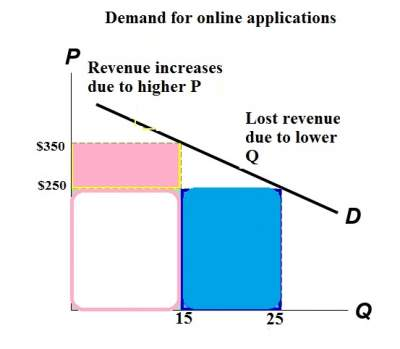On the other hand,
• If demand is inelastic, then price elasticity of demand < 1
Percentage of change in Q < Percentage of change in P
• The fall in revenue from lower Q is less than the increase in revenue from higher P, hence revenue rises.
• For example, let's assume that Q only falls to 20 (instead of 15) when P is increased to \$350.
•  Elastic demand. In this case, elasticity = 0.67 If P = \$250, Q = 25 and revenue = \$5000 If P = \$350, Q = 20 and revenue = \$7000 When D is inelastic, a price increase causes revenue to rise.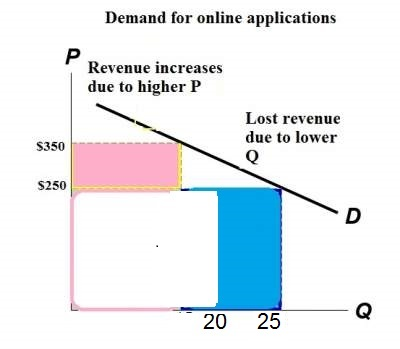### Exercises

Exercise-1 on Elasticity and Expenditure/Revenue

Check your answers here: Solution to the Exercise-1 on Elasticity and Expenditure/Revenue

Exercise-2 on Elasticity and Expenditure/Revenue

Check your answers here: Solution to the Exercise-2 on Elasticity and Expenditure/Revenue